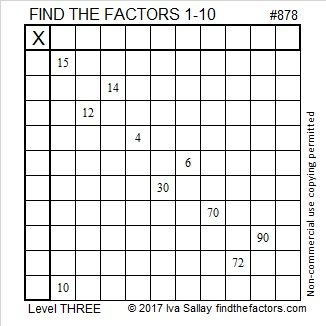# 878 and Level 3

218 + 219 + 220 + 221 = 878; that’s the sum of four consecutive numbers.

438 + 440 = 878; that’s the sum of two consecutive even numbers.

878 is a palindrome in base 10 but not in any of the other bases from 2 to 36.Print the puzzles or type the solution on this excel file: 10-factors-875-885

• 878 is a composite number.
• Prime factorization: 878 = 2 × 439
• The exponents in the prime factorization are 1 and 1. Adding one to each and multiplying we get (1 + 1)(1 + 1) = 2 × 2 = 4. Therefore 878 has exactly 4 factors.
• Factors of 878: 1, 2, 439, 878
• Factor pairs: 878 = 1 × 878 or 2 × 439
• 878 has no square factors that allow its square root to be simplified. √878 ≈ 29.63106This site uses Akismet to reduce spam. Learn how your comment data is processed.